Latest Teaching jobs   »   Mensuration-3D: Download Mathematics Study Notes Free...

# Mensuration-3D: Download Mathematics Study Notes Free PDF For REET/UTET Exam

`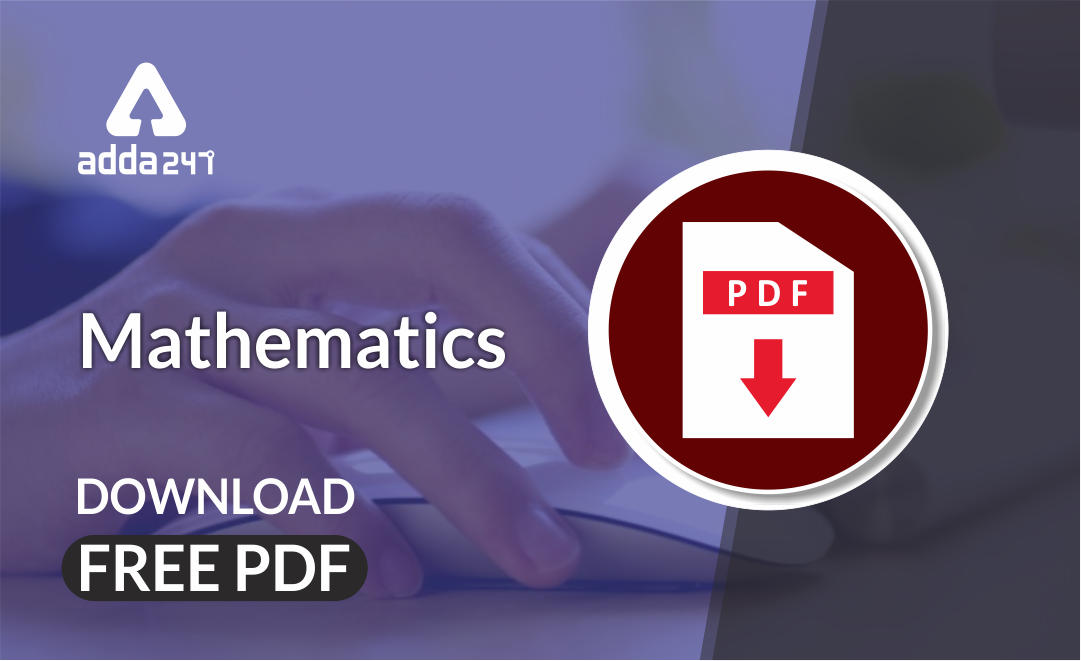`

Mathematics is an equally important section for  REETMPTET & DSSSB Exams and has even more abundant importance in some other exams conducted by central or state govt. Generally, there are questions asked related to basic concepts and formulas of the Mensuration.

To let you make the most of Mathematics section, we are providing important facts related to the Mensuration 3-D. At least 2-3 questions are asked from this topic in most of the teaching exams. We wish you all the best of luck to come over the fear of the Mathematics section.

How to Overcome Exam Fever, Especially When You Fear Maths

## Study Notes on Mensuration – 3D

3D Shapes: All 3D shapes can be described in terms of their faces, vertices and edges.

Face: A flat or curved surface

Edge: Line where 2 faces meet

Vertex: A point where 3 or more edges meet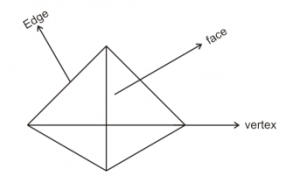• Cuboid: It is a solid figure which has 6 regular faces, 12 edges, 8 vertices and 4 diagonals.

Let length = L, Breadth = b and height = h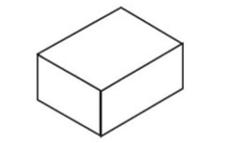Volume = (l×b×h)

Total surface Area= 2(lb+bh+hl)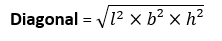Ex.  The length, breadth and height of a cuboid are in the ratio 5:6:8 and its volume is 1920 cm³. The total surface area of cuboid is: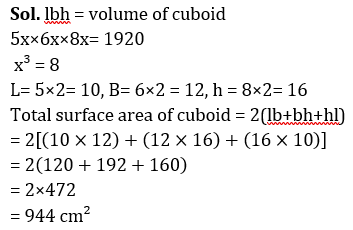Get free Study material for REET Exam

• Cube: A cube has 6 equal faces, 12 equal edges, 8 vertices and 4 equal diagonals. Let edge = a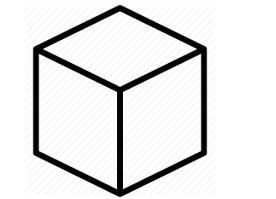Volume = a³

Total surface area = 6a²

Lateral surface area = 4a²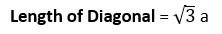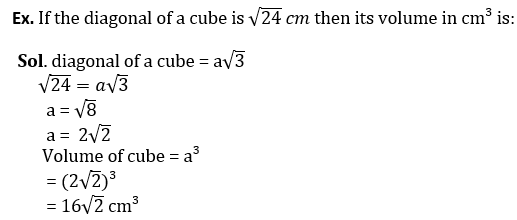• Cylinder: A right circular cylinder is a cylinder whose base is a circle and whose elements are perpendicular to its base. Let radius = r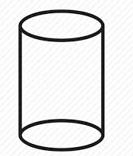Height = h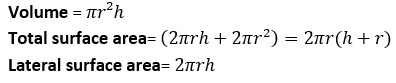Ex.  The radius of a cylinder is 28cm and its height is 54cm. Its volume is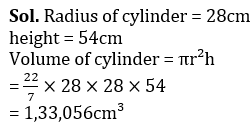Practice REET Previous Year Maths Quiz For REET Exam Here

• Cone: A right circular cone is one whose axis is perpendicular to the plane of the base. Let radius = r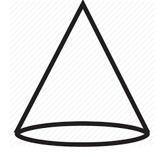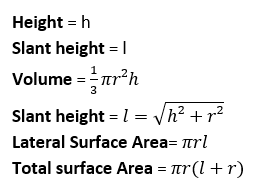Ex. Curved Surface area of a cone whose volume is 4,224 cm³ and radius is 24cm, is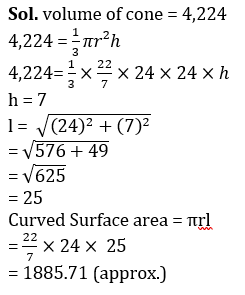• Frustum of a cone: When a cone is cut by a plane parallel to the base of the cone then the portion between the plane and the base is called the frustum of the cone.

Let, radius of base= R, radius of top =r and height =h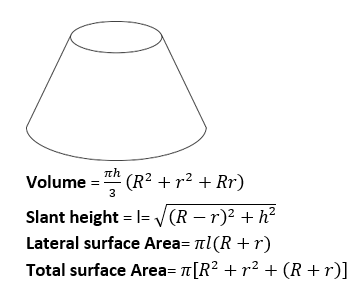Mathematics Study Notes For All Teaching Exams

• Sphere: A sphere is a solid bounded by a closed surface every point of which is equidistant from a fixed point called the center. Let the radius = r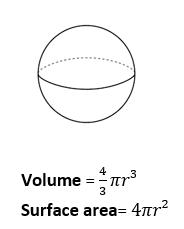Ex. The radius of solid metallic sphere is 8cm, find the volume of the sphere.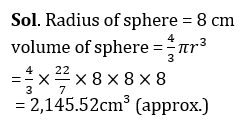• Hemisphere: A plane through the center of the sphere cuts it into two equal parts. Each part is called Hemisphere. Let the radius =r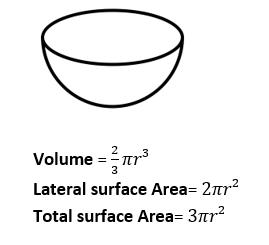Ex.  The radius of hemispherical bowl is 27cm. find the volume of the bowl.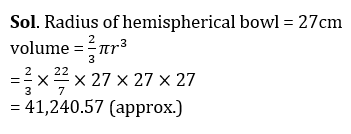• Pyramid: A pyramid is made by connecting a base to an apex. There are many types of pyramid and they are named after the shape of their base.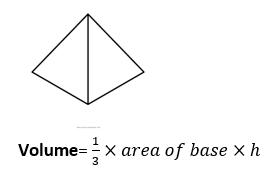Total surface area= Area of base + Area of each of the lateral faces

Ex. Area of base of a pyramid is 89 cm² and height is 9 cm then its volume (in cm³) is: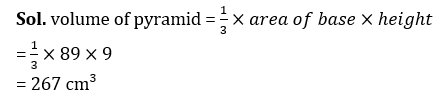• Prism: A prism is a solid object with identical ends and flat faces.

Volume = area of base × height

Total surface Area = 2(area of base) + (perimeter of base × height)

Curved surface Area = perimeter of base × height

### Download Important Mensuration 3D Notes PDF (Hindi)

Sharing is caring!

Thank You, Your details have been submitted we will get back to you.
•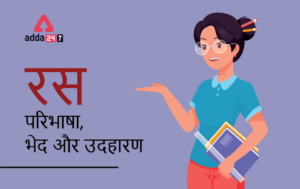रस - परिभाषा, भे�...
•Learning Disabilities : Math Dyslexia, D...
•Flander's Interaction Category System (F...
•Kothari Commission (1964-66) कोठ�...
•विशेषण- परिभाष�...
•Phases of Teaching - Stages of Teaching ...